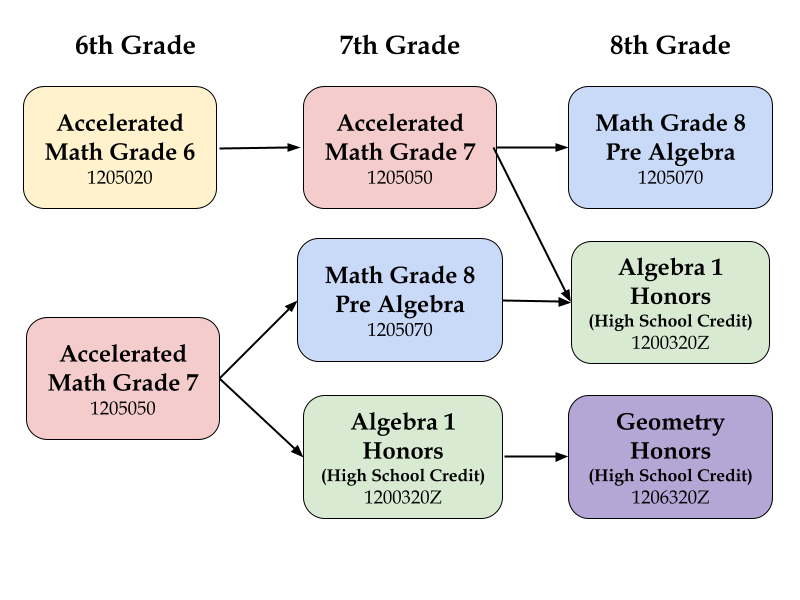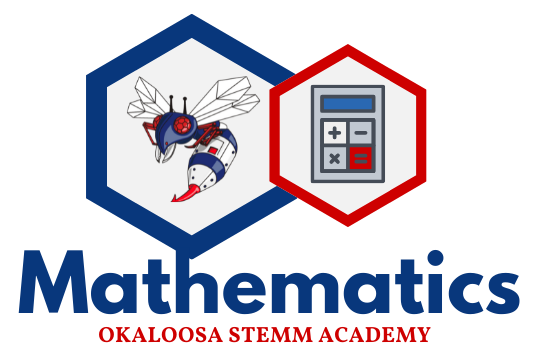# Math Department

Through subject acceleration, our 6th graders typically take Accelerated Math Grade 7, which means they are going straight from fifth-grade math into seventh-grade advanced math. Our 7th graders then progress to Algebra, and our 8th graders finish with Geometry. Rarely, we have a group of students who progress along an alternate track, taking Accelerated Math Grade 6 or Grade 8 PreAlgebra.

Mathematics Course ProgressionAlgebra I for High School Credit
Middle school students enrolled in Algebra I must take the Algebra I EOC assessment, which constitutes 30% of the student’s final grade, AND pass the course to earn high school Algebra I credit.

A middle school student is not required to earn a passing score on the Algebra I EOC assessment in order to earn high school credit to be promoted to high school.

Middle school students enrolled in high school Algebra I must pass the statewide EOC assessment to qualify for a standard high school diploma. However, if the student does not pass the EOC assessment in middle school, the student will have multiple opportunities in high school to retake the course and/or the assessment.

Geometry for High School Credit
Middle school students enrolled in high school Geometry must take the Geometry EOC assessment, which constitutes 30% of the student’s final course grade and earn a passing grade in the course in order to qualify for a standard high school diploma scholar designation. The final course grade is included in the high school GPA.# Mathematics Course Descriptions

### 1205020 M/J Accelerated Mathematics Grade 6

In this Grade 6 Advanced Mathematics course, instructional time should focus on six critical areas: (1) connecting ratio and rate to whole number multiplication and division and using concepts of ratio and rate to solve problems; (2) completing understanding of division of fractions and extending the notion of number to the system of rational numbers, which includes negative numbers; (3) writing, interpreting, and using expressions and equations; (4) developing understanding of statistical thinking; (5) developing understanding of and applying proportional relationships; and (6) developing understanding of operations with rational numbers and working with expressions and linear equations.

### 1205050 M/J Accelerated Mathematics Grade 7

In this Grade 7 Advanced Mathematics course, instructional time should focus on five critical area: (1) solving problems involving scale drawings and informal geometric constructions, and working with two- and three-dimensional shapes to solve problems involving area, surface area, and volume; (2) drawing inferences about populations based on samples; (3) formulating and reasoning about expressions and equations, including modeling an association in bivariate data with a linear equation, and solving linear equations and systems of linear equations; (4) grasping the concept of a function and using functions to describe quantitative relationships; and (5) analyzing two- and three-dimensional space and figures using distance, angle, similarity, and congruence, and understanding and applying the Pythagorean Theorem.

### 1200320Z Algebra I Honors

High School Credit: 1 Credit

Course Weight: 4.5

Prerequisite(s): FSA Math Level 4 or Higher. An “A-B” average in M/J Math 2 Advanced, Math Prealgebra or M/J Math 3 Advanced is highly recommended for highest potential for academic success.

This course gives a rigorous in-depth study of algebra and algebraic concepts and processes that can be used to solve a variety of real-world mathematical problems. Topics will include the language of algebra, solving equations with one and two variables, polynomials, quadratics, exponents, statistics, factoring functions, and graphing. At the completion of this course, all students will take the state mandated EOC exam which counts 30% of the student’s final grade. Passing the Algebra 1 EOC is required for graduation.